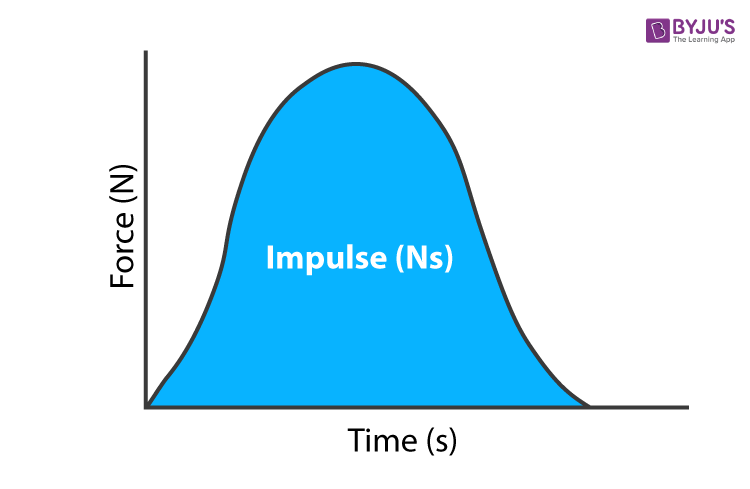Jet Set Go! All about Aeroplanes Jet Set Go! All about Aeroplanes

# Impulse Units

## What Is Impulse?

The term Impulse is used to refer to fast-acting force or “impact”, Thus impulse can be defined as “The sudden force acting on an object for a short interval of time”. Conventionally represented by “J”. Sometimes by “imp” and expressed in “N.s”.

The impulse, when plotted against time, the graph of unit impulse is Gaussian in nature. The graph looks like this-In classical mechanics, an impulse is expressed as an integral of a resultant force over an interval of time on which it acts. It is a vector quantity. Which implies it has direction along with magnitude. We know that force is a vector quantity, Impulse arise as a result of force, hence this vector quantity follow the same direction as that of force.

An object in the presence of any force, accelerate or change the velocity. The same force acting on the same body for a long duration of time will cause a greater change in its linear momentum as compared to the same for a short duration. Similarly, small force applied for a long duration will cause the same change in momentum as a large force – Impulse force for a short duration.

Change in momentum is equal to the product of the average force and time duration. Mathematically written as

$$\begin{array}{l}J=F_{avg}\left ( t_{2}-t_{1} \right )\end{array}$$

We know that Impulse is the integral of force F over time:

$$\begin{array}{l}J=\int Fdt\end{array}$$

### Impulse – Momentum Theorem

Impulse J produced between the time interval t1 and t2 is given by-

$$\begin{array}{l}J=\int_{t_{1}}^{t_{2}}Fdt \,\, \textup{—–(1)}\end{array}$$

Where F is the resultant force acting in the time interval t1 and t2

Now by applying Newton’s second law of motion, we can relate Force F and momentum P as –

$$\begin{array}{l}F=\frac{dP}{dt}\end{array}$$

Thus, substituting it in equation (1), we get-

$$\begin{array}{l}J=\int_{t_{1}}^{t_{2}}\frac{dP}{dt}dt\end{array}$$
$$\begin{array}{l}\Rightarrow J=\int_{p_{1}}^{p_{2}}dP\end{array}$$
$$\begin{array}{l}J=P_{2}-P_{1}\end{array}$$
$$\begin{array}{l}J=\Delta P\end{array}$$

“Impulse acting on a body changes equal amount of linear momentum in the same direction.”

This equivalence of Impulse and Momentum is called as Impulse-Momentum Theorem.

Thus, Impulse can be expressed as the change in momentum of an object to which force is applied. When the mass of the object is constant, Then impulse can be written as –

$$\begin{array}{l}J=\int_{t_{1}}^{t_{2}}Fdt=\Delta P=mv_{2}-mv_{1}\end{array}$$

Where,

• J is the Impulse
• F is Force applied
• m is the mass of the object
• v1 and v2 are the initial and final velocity respectively during the presence of force
• t1 and t2 are the beginning and end time respectively during the presence of force

Read More: Newton’s Second Law of Motion

## Impulse Units

The units of impulse can be derived using the Impulse-momentum theorem. Impulse is equivalent to a change in momentum, This can be mathematically written as-

$$\begin{array}{l}\sum\vec{F}\Delta t=m\Delta\vec{v}\end{array}$$

The left-hand side of the equation clearly says that Force is multiplied by time to give impulse. According to SI system unit of Impulse will be newton second or “N.m”.

Here newton (N) is a derived unit/compound unit. Using Newton’s second law, the force can be written as the product of mass and square of velocity, that is,

$$\begin{array}{l}F=ma=mv^{2}\end{array}$$

Expressing the above equation in terms of their SI units, we get-

$$\begin{array}{l}N=kg\frac{m}{s^{2}}\end{array}$$

On multiplying both sides by a second we get-

$$\begin{array}{l}N.s=kg\frac{m}{s^{2}}.s\end{array}$$
$$\begin{array}{l}=kg\frac{m}{s}\end{array}$$
$$\begin{array}{l}=kgm/s\end{array}$$

Thus, it has the same units and dimensions as momentum.

 SI unit N.s Dimensional formula [MLT-1] English engineering unit lbf.s Dimensionally equivalent unit of momentum kg.m.s-1

### Real-life instances

We come across impulse at various situations of our day-to-day life. Some of such scenarios are listed below-

• Airbags in cars are designed on the basis of the principle of Impulse
• Tossing the coin
• Hitting golf ball
• Batsman hitting the ball during the cricket match
• Kick-starting the motorbike etc.

Physics-related articles:

## Frequently Asked Questions – FAQs

### Define impulse.

The sudden force acting on an object for a short time interval is known as an impulse.

### Impulse is a vector or scalar quantity.

Impulse is a vector quantity.

### What is the SI unit of impulse?

The SI unit of impulse is Newton-seconds (Ns) or kg m/s.

### Is hitting golf ball an example of impulse?

Yes, hitting a golf ball is an example of impulse in various situations of our day-to-day life.

### State true or false: Impulse acting on a body changes an equal amount of linear momentum in the same direction.

True.

We hope you have understood the concept. Also, download “BYJU’S – The Learning App” for interactive videos and academic assistance.

Test your knowledge on Impulse units